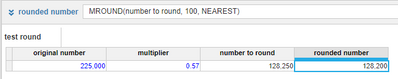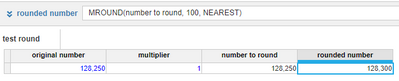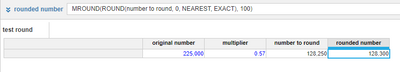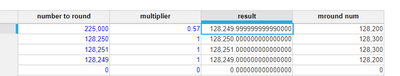# MROUND showing inconsistent resultsHi, I am trying to use MROUND formula to round to the nearest 100.

However, there is some inconsistency that I spotted.

original number * multiplier = number to round

Example 1:Example 2:Why are the output number different when the "number to round" is the same?

I want it to round to the nearest 100. If there number is just nice halfway in between, it should round up.

How should I apply the formula to round up for example 1?

Thanks,

Peggy

Tagged:

•Thanks for the quick reply both! But isn't 225,000 * 0.57 a perfect whole number of 128,250? Why is it not a whole number in anaplan? 😕

Anyway I found the solution to just apply a ROUND formula first before MROUND so that solves it now.•Hi, I think that's because the underlying number is actually "128249.99999999999" and when you round it to the nearest 100, it will give 128200 which is nearer than 128300. In the second case, there are no decimals and the nearest value would be different. Hope it helps!•Hi,

When you select a cell, Anaplan shows the exact value at the top right of your module (in front of formula tab). I think the first value would is 128249.999 and the second is 128250 and that is why first one is rounding off to a 128200. Just a hunch. Please check

Edit: In second case, when the value lies exactly in the middle, Anaplan rounds up towards positive infinity and thus it rounds up to 128300

•